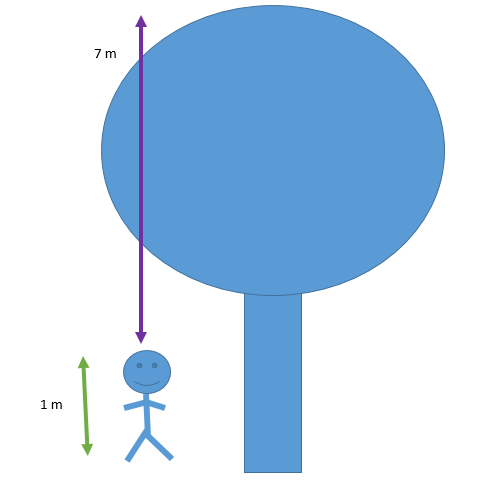QuestionAnswers

# I am 1 meter tall and the top of the tree I am standing under reaches 7 meter higher than me. How high is the tree?A) 5 mB) 6 mC) 7 mD) 8 mHint: We will first try and depict what the question wants to convey and draw a basic rough diagram to see the same and then we will see that summing up both the numbers will lead us to the height of the tree.

We are given that the person is 1 m tall and he / she is standing under a tree which goes 7 m above their head. Let us try to depict the same with a diagram.We see that the person is 1 meter tall and the height of the tree above him is 7 meters.
We obviously know that both the person and tree will be standing on the ground only. So, if we clearly observe the height of the tree, the total would be the sum of the height of the tree above the person and the height of the person himself / herself.
So, height of tree will be given by: Height of tree above person + Height of person
Hence, the height of the tree = 7 m + 1 m = 8 m.
Hence, the answer is 8 meters.

Hence, the correct option is (D).

Note: The students must make a rough diagram to see the things clearly. If they want to they can even draw lines for both the person and tree as well instead of a clearer diagram. They just need the diagram to understand the given conditions.
Addition of 0 to any number does not change anything.
Addition of any number to itself will always result in zero. In simpler words for all real numbers a, a + (-a) = 0 always.
View Notes
What is the full form of AM and PMCBSE Class 6 Maths Chapter 12 - Ratio and Proportion FormulasI Love My Family EssayClass 6 IMO Maths Olympiad Sample Question Paper - 1IMO Sample Papers for Class 6The Idea of TimeNCO Olympiad Sample Paper 1 for Class 6Table of 6 - Multiplication Table of 6IGKO Olympiad Sample Paper 1 for Class 6NSO Science Olympiad Sample Paper 1 for Class 6Maths Question Paper for CBSE Class 12 - 2016 Set 1 EMaths Question Paper for CBSE Class 12 - 2016 Set 1 SMaths Question Paper for CBSE Class 12 - 2016 Set 1 CMaths Question Paper for CBSE Class 12 - 2016 Set 1 NCBSE Class 10 English Language and Literature Question Paper with SolutionsEnglish Language and Literature Question Paper for CBSE Class 10 - 2012CBSE Class 12 Maths Question Paper 2020English Language and Literature Question Paper for CBSE Class 10 - 2010English Language and Literature Question Paper for CBSE Class 10 - 2011Previous Year Question Paper of CBSE Class 10 EnglishNCERT Solutions for Class 6 English Honeysuckle Chapter 6 - Who I AmRD Sharma Class 6 Solutions Chapter 1 - Knowing Our Numbers (Ex 1.6) Exercise 1.6RS Aggarwal Solutions Class 6 Chapter-1 Number System (Ex 1F) Exercise 1.6RS Aggarwal Solutions Class 6 Chapter-6 Simplification (Ex 6A) Exercise 6.1RD Sharma Class 6 Solutions Chapter 6 - Fractions (Ex 6.1) Exercise 6.1NCERT Solutions for Class 6 Maths Chapter 6 Integers (Ex 6.1) Exercise 6.1RS Aggarwal Solutions Class 7 Chapter-6 Algebraic Expressions (Ex 6A) Exercise 6.1RS Aggarwal Solutions Class 8 Chapter-6 Operations on Algebraic Expressions (Ex 6A) Exercise 6.1RD Sharma Class 9 Solutions Chapter 6 - Factorization of Polynomials (Ex 6.1) Exercise 6.1RD Sharma Class 6 Solutions Chapter 1 - Knowing Our Numbers (Ex 1.1) Exercise 1.1